# Transformation: Translation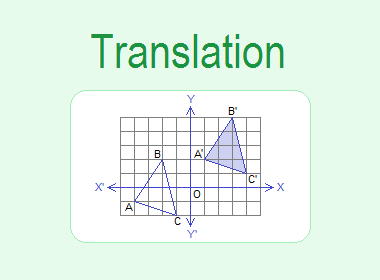The Translation is a transformation in which each point of the given object is displaced through definite distance and direction. The displacement is defined by a translation vector (a, b).

********************

10 Math Problems officially announces the release of Quick Math Solver, an Android App on the Google Play Store for students around the world.

********************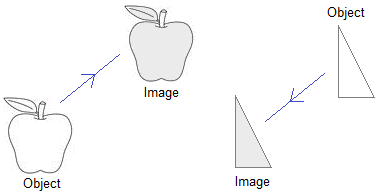The following are the properties of translation:

1.   The object and the image under the translation are congruent.

2.   The lines joining any point of the object with its corresponding image are parallel and equal.

Example 1: Find the image of ΔABC under the translation through translation vector PQ.Solution:

To get the image of ΔABC under the translation vector PQ draw the lines through A, B, and C which are parallel and equal to PQ in the same direction to get the images of A, B, and C and join them to get ΔA’B’C’.## Translation Using Co-ordinates

The image of the geometrical figures under the translation through certain translation vector can be obtained with the help of co-ordinates.

### Translation Through Translation Vector T = (a, b)

Let A(2, 1) be a point and T = (2, 3) be the translation vector. To translate A(2, 1) through translation vector T = (2, 3), it has to move 2 units right and then 3 units up which is the point (4, 4) as shown in the graph given below.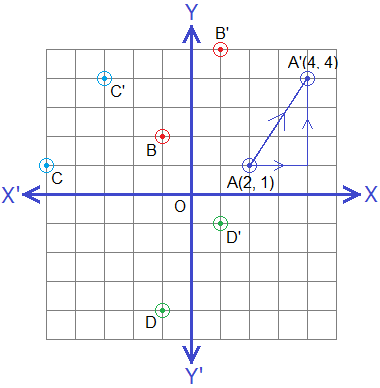Hence, A’(4, 4) is the image of the point A(2, 1) under the translation vector T = AA’ = (2, 3).Let us see the following table of some other points on the same graph and their corresponding images under the translation through translation vector T = (2, 3).From the above table, we can see that the image of any point under the translation through translation vector (a, b) is obtained by adding a to x-coordinate and b to y-coordinate of the given point.### Worked Out Examples

Example 2: If A(-4, -4), B(-2, -1) and C(-1, -5) are the vertices of a triangle ABC. Find the co-ordinates of the image of ΔABC under the translation T =(6, 5). Draw ΔABC and its image on the same graph paper.

Solution:

As A(-4, -4), B(-2, -1) and C(-1, -5) are the vertices of ΔABC, the co-ordinates of the vertices of image of ΔABC can be obtained by using the formula as below:Drawing ΔABC and ΔA’B’C’ on the same graph paper we have figure as shown.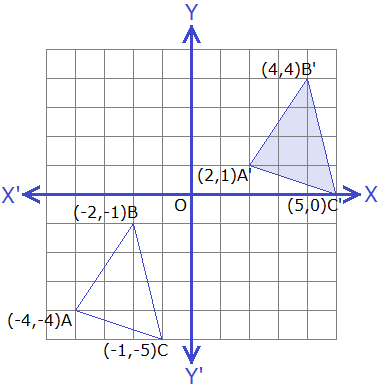Example 3: A(-4, 2), B(0, 1) and C(-2, -3) are the vertices of ΔABC. If A’(-1, 6) be the image under the translation of vertex A. Find the images B’ and C’ of B and C under the same translation.

Solution:

Here, as the image of A(-4, 2) under the translation is A’(-1, 6),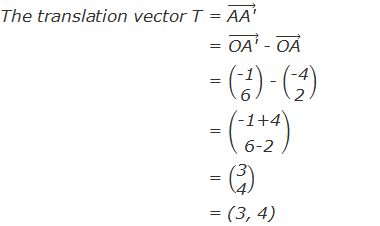Now, under the same translation, the image of B(0, 1) is B’(0+3, 1+4) i.e. B’(3, 5) and the image of C(-2, -3) is C’(-2+3, -3+4) i.e. C’(1, 1).

Example 4: Translate a point A(2, 7) under translation T1 = (4, -3) to the point A’. Translate A’ to A’’ under another translation T2 =(2, 4). Find the translation vector which translates A to A’’.

Solution:Now, let T be the translation vector which translates A(2, 7) to A’’(8, 8).Example 5: Translate the point M(4, -5) by the translation vector T = (-3, 4) and find the image point M’ and write down the translation vector which maps M’ to M.

Solution: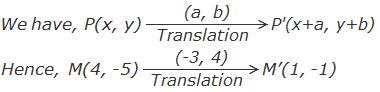Let T’ = (a, b) be the translation vector which maps M’(1, -1) back to M(4, -5). Then,Therefore,

1+a = 4

or, a = 4 – 1

or, a = 3

And,

-1+b = -5

or, b = -5 + 1

or, b = -4

The required translation vector is (3, -4) which is called the inverse of T and written as T-1.

i.e.    If T = (a, b) then T-1 = (-a, -b).

#### Do you have any questions regarding the translation?

You can ask your questions or problems here in the comment section below.

1.Am vary happy for your lesson

2.Great and straight forward lesson. Making Translation much easier
Thank you.

3.A point p is translated by vector (3,4)to point p(6,5).find the coordinates of p

1.Coordinates of P = (6-3, 5-4) = (3, 1)